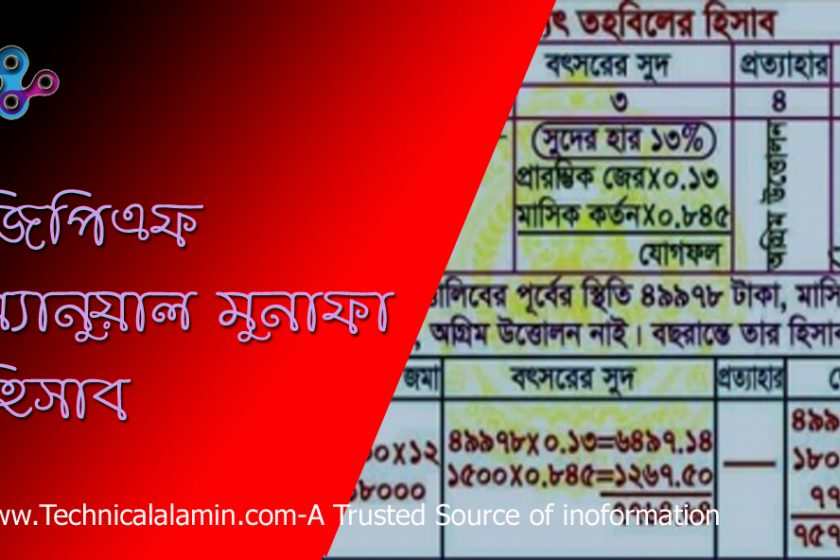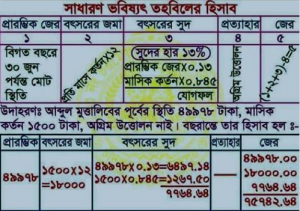# GPF Profit Calculation । জিপিএফ মুনাফা বের করার নিয়ম

GPF profit calculation – Today i will show you how to calculate gpf interest or profit alone manually. This is very simple method if you know simple math. imagine GPF profit rate is 13%.

First say, your beginning Balance of your gpf 00 taka. You are planning to subscribe 1000 taka per month, In a year your deposit will be 12 thousand taka only. Interest and annual profit will be at A*13/2*R (Interest Rate).

Interest Calculation: A*13/2*R (1000*13/2*13%) = 845 Taka. Your yearly total interest will be 845 taka and Total amount will be 12,845 taka only. After getting online or offline gpf slip pls calculate your interest manually to avoid miscalculation.

## GPF Calculation is now very easy process / You can calculate GPF interest calculation

Every year gpf slip is being distributed / Match your calculation to your gpf slipCaption: GPF calculation System / GPF calculation process which is used in accounts office